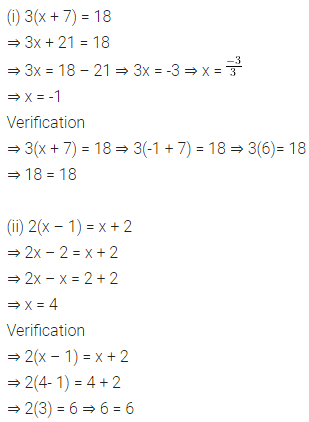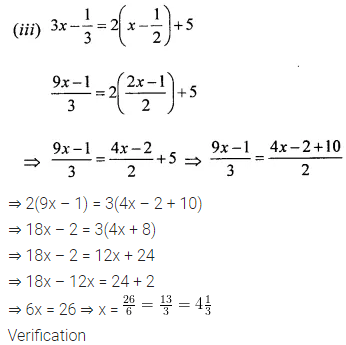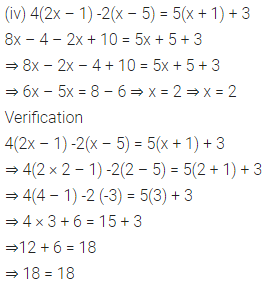# ML Aggarwal Class 6 Solutions for ICSE Maths Chapter 9 Algebra Ex 9.5

## ML Aggarwal Class 6 Solutions for ICSE Maths Chapter 9 Algebra Ex 9.5

Question 1.
State which of the following are equations with a variable. In case of an equation with a variable, identify the variable.
(i) 17 + x = 5
(ii) 2b – 3 = 7
(iii) (y – 7) > 5
(iv) $$\frac{9}{3}=3$$
(v) 7 × 3 – 19 = 2
(vi) 5 × 4 – 8 = 31
(vii) 2p < 15
(viii) 7 = 11 × 5 – 12 × 4
(ix) $$\frac{3}{2} q=5$$
Solution: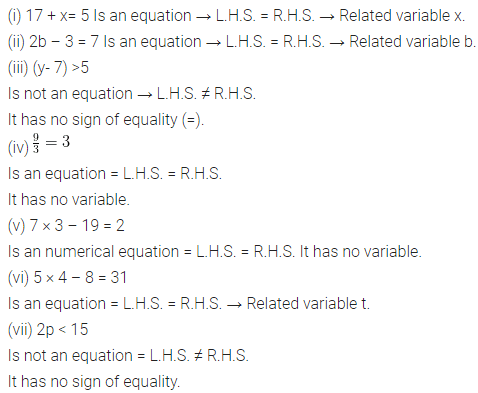Question 2.
Solve each of the following equations :
(i) x + 6 = 8
(ii) 2 – x = 5
(iii) 4x = -6
(iv) $$\frac{x}{2}=5$$
(v) 2y – 3 = 2
(vi) 4 – 5y = 2
Solution:Question 3.
Solve the following linear equations:
(i) 5(x + 1) = 25
(ii)2(3x – 1) = 10
(iii) $$\frac{3 x-1}{4}=11$$
Solution: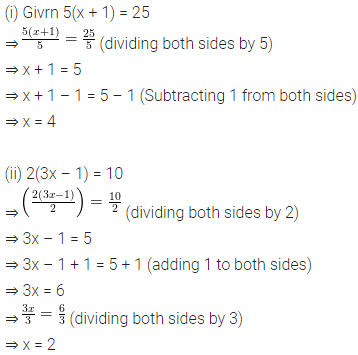Question 4.
Solve the following linear equations: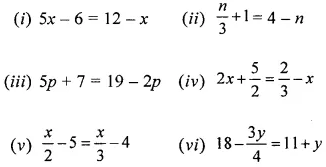Solution: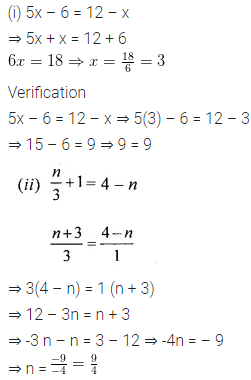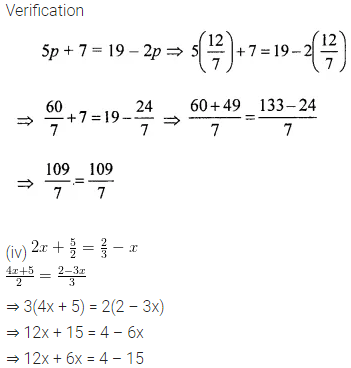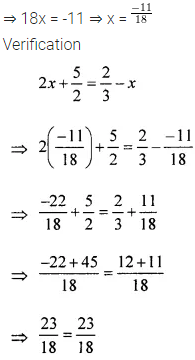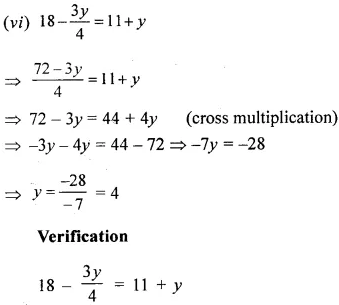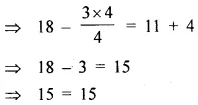Question 5.
(iii) $$3 x-\frac{1}{3}=2\left(x-\frac{1}{2}\right)+5$$